# Plotting¶

How can we plot mathematical objects in sagemath?

A directed graph consists of vertices and edges. Edges are directed, thus have a source and destination. We specify an edge as some id (e.g. 1) associated with a list of ids it points to (e.g. [2, 3]):

In :
d = {1: [2, 3], 2: , 3: [5, 6], 4: , 5: , 6: [6, 7], 7: }
g = DiGraph(d)
g.plot()

Out: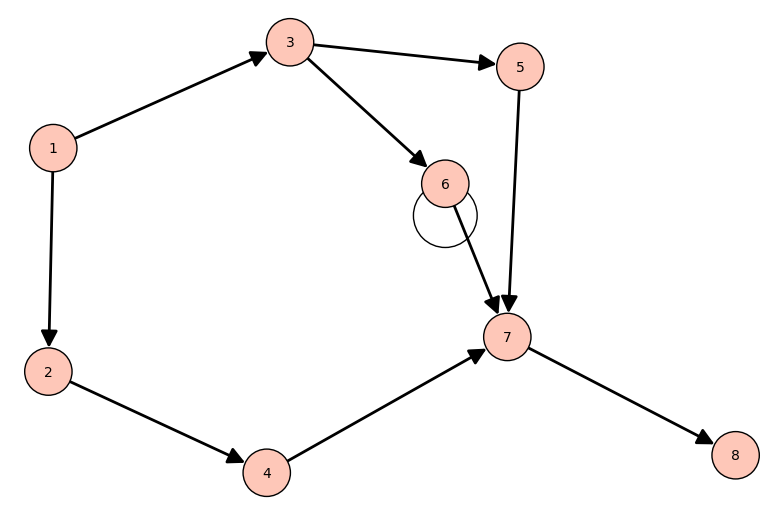One typical problem is shown here: we plot a function on a given domain (here: from ‘-5’ to ‘5’), but the function is only defined on a smaller domain (namely ‘0’ to ‘5’):

In :
plot(sqrt(x), x, -5, 5)

verbose 0 (3763: plot.py, generate_plot_points) WARNING: When plotting, failed to evaluate function at 100 points.
verbose 0 (3763: plot.py, generate_plot_points) Last error message: 'math domain error'

Out: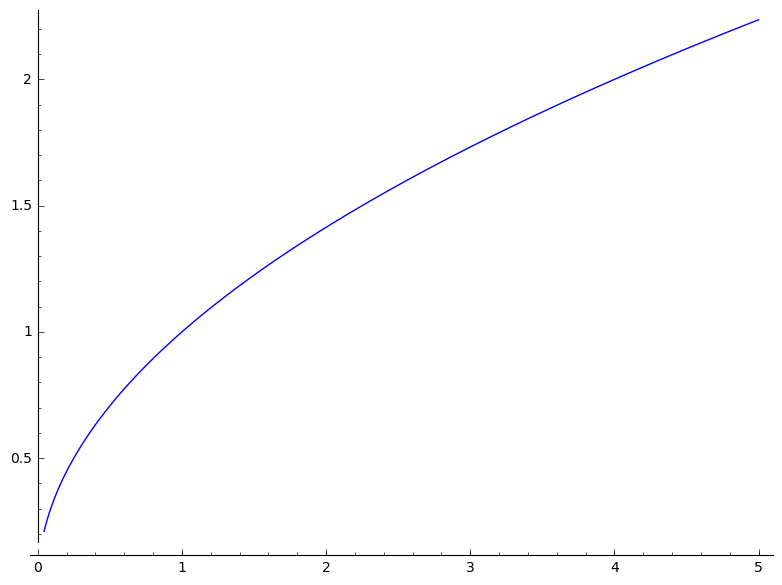We can provide keyword-argument ‘detect_poles’, but this will often fail (as pole detection is mathematically difficult). However, I present one function which shows the functionality nicely:

In :
plot(tan(2*x), x, -pi, pi, ymin=-5, ymax=5, detect_poles='show')

Out: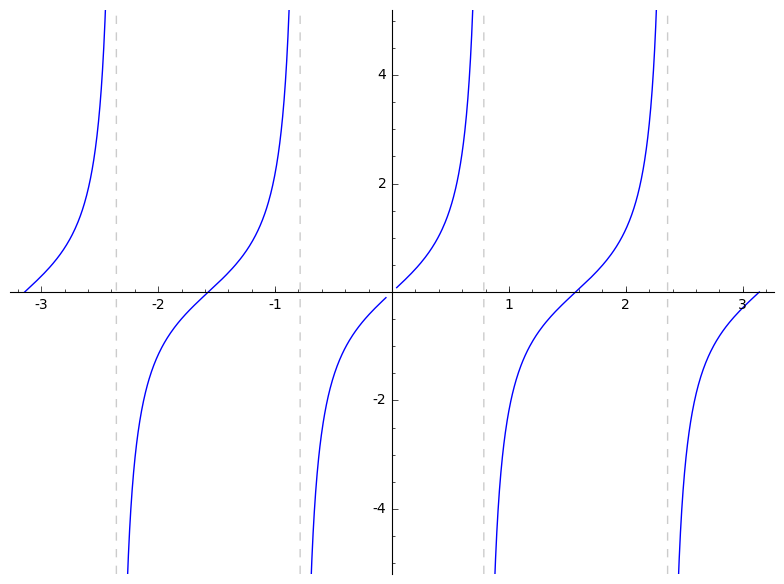The actual solution is to define a piecewise function (mathematically this extends the domain of the partial function). Here, we slice the domain into to intervals [-5, 0] and (0, 5). Recognize that lists are used to denote closed intervals (matches mathematical notation) and tuples are used for open intervals (matches mathematical notation). ‘0’ denotes the constant zero function:

In :
f = piecewise([
([-5, 0], 0),       # f(x) = 0        on [-5, 0]
((0, 5), sqrt(x))   # f(x) = sqrt(x)  on (0, 5)
])

plot(f)

Out: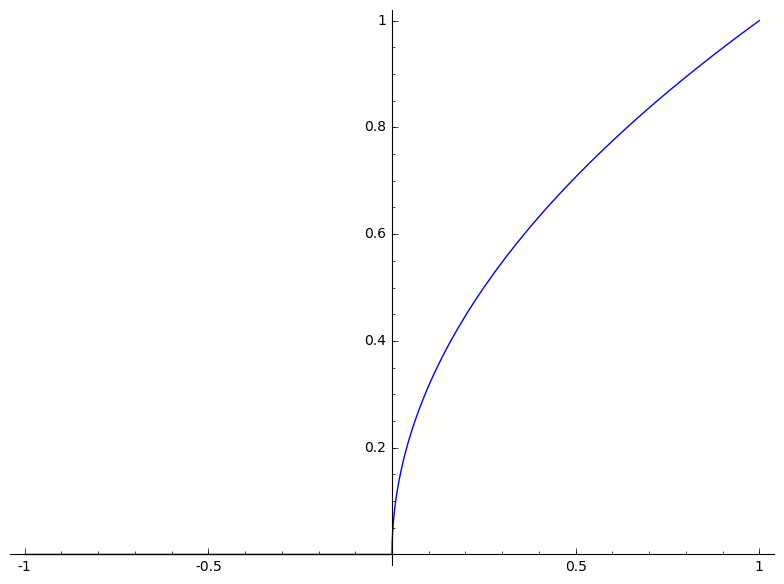How about mixed intervals? Use the RealSet methods to retrieve subsets of the real line:

In :
f = piecewise([
([-5, 0], 0),                         # f(x) = 0        on [-5, 0]
(RealSet.open_closed(0,5), sqrt(x))   # f(x) = sqrt(x)  on (0, 5]
])

plot(f)

Out: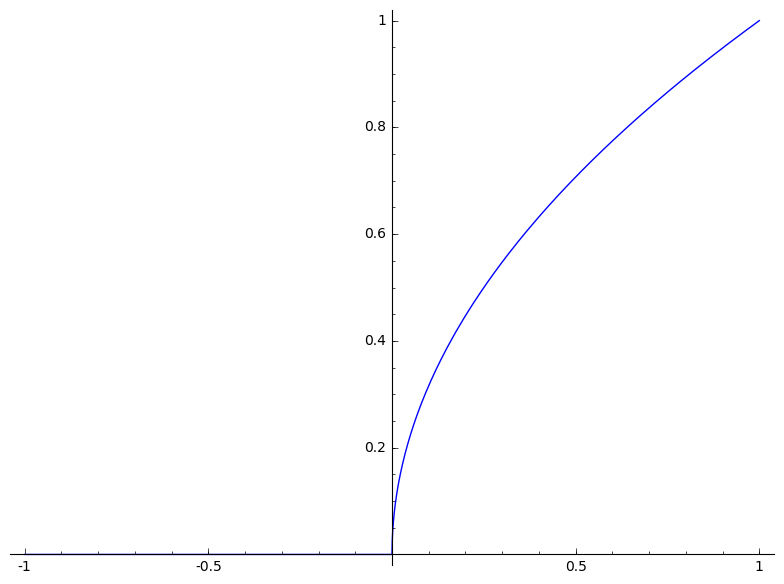3D plots also look cool. Here a function takes x- and y-values and returns the corresponding z-value:

In :
f(x, y) = sin(x) * cos(y)
plot3d(f, (x, -pi, pi), (y, -pi, pi))

Out:

Parametric plots are also possible. In case of parametric plots, the input argument(s) is not a dimension itself, but iterates over a domain. The given function returns a tuple specifying all values in all dimensions. Here Neil's parabola is used as an example:

In :
var('x')
parametric_plot((x^2, x^3), (x, -1, 1))

Out: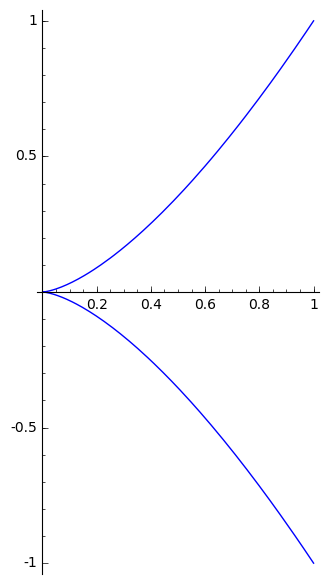One super-cool plot from the documentation are these interwoven tori:

In :
# source: https://doc.sagemath.org/html/en/reference/plot3d/sage/plot/plot3d/parametric_plot3d.html#sage.plot.plot3d.parametric_plot3d.parametric_plot3d
u, v = var('u,v')
f1 = (4 + (3 + cos(v)) * sin(u), 4 + (3 + cos(v)) * cos(u), 4 + sin(v))
f2 = (8 + (3 + cos(v)) * cos(u), 3 + sin(v), 4 + (3 + cos(v)) * sin(u))
p1 = parametric_plot3d(f1, (u, 0, 2 * pi), (v, 0, 2 * pi), texture="red")
p2 = parametric_plot3d(f2, (u, 0, 2 * pi), (v, 0, 2 * pi), texture="blue")
p1 + p2

Out:

This polar(-coordinate) plot from the documentation also looks super-nice:

In :
# http://doc.sagemath.org/html/en/reference/plotting/sage/plot/plot.html#sage.plot.plot.polar_plot
polar_plot([(1.2 + k * 0.2) * log(x) for k in range(6)], 1, 3 * pi, fill={0: , 2: , 4: })

Out: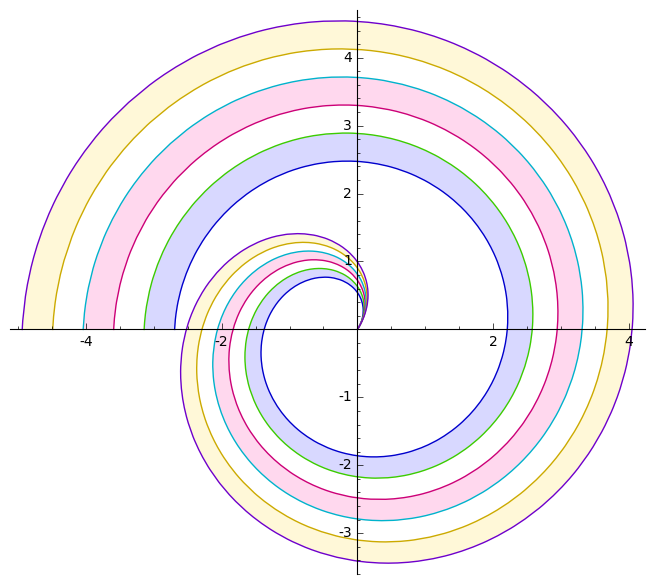Finally, you can also make bar charts, but recognize the issues:

• the legend shows too narrow color boxes
• It is not possible to annotate all bars along the x-axis

Personally, I concluded that this is one area where sagemath is impractical in terms of plotting.

In :
# data source: deleted 'Grazer Linuxtage' DE-Wikipedia article
Unknown = 0
lectures = [17, 31, 23, 28, 29, 29, 29, 29, 35, 34, 27, 33, 40, 42, 35, 37, 45]
workshops = [Unknown, 3, 6, 5, 4, Unknown, Unknown, 1, 1, 5, 3, 12, 13, 12, 8, 12, 17]

fig = plot([], title="Number of accepted GLT submissions", axes_labels=['years', '#'])
fig += bar_chart(lectures, color='r', legend_label='lectures')
fig += bar_chart(workshops, color='b', legend_label='workshops')
fig.show(show_legend=True, legend_loc="upper center")